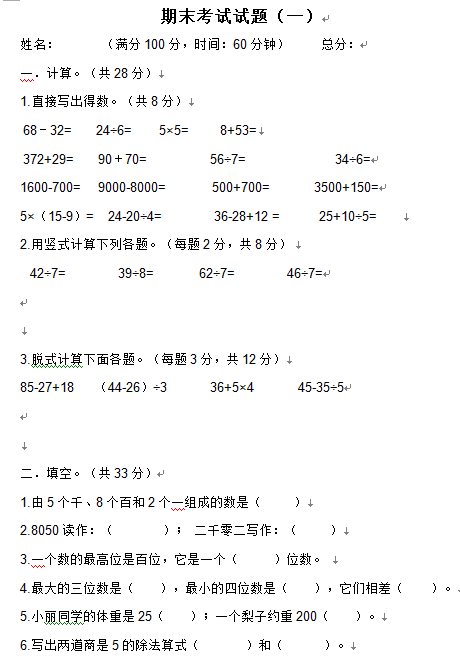1.直接写出得数。（共8分）
68－32=      24÷6=       5×5=        8+53=
372+29=      90＋70=            56÷7=              34÷6=

1600-700=    9000-8000=       500+700=       3500+150=

5×（15-9）=    24-20÷4=          36-28+12 =      25+10÷5=

【DOC文档4页】小学二年级数学下册期末考试试题(一)A4电子版资料_可直接打印_会员免费下载##### 评论信息